## Saturday, January 05, 2019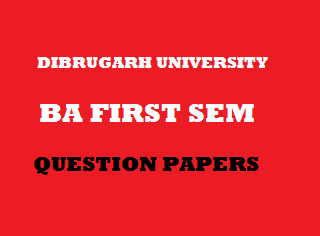2014
(November)
MATHEMATICS
(General)
Course: 101
[(a) Classical Algebra, (b) Trigonometry, (c) Vector Calculus]
Full Marks: 80
Pass Marks: 32
Time: 3 hours
The figures in the margin indicate full marks for the question
GROUP – A
(Classical Algebra)

1. (a) Write the range set of the sequence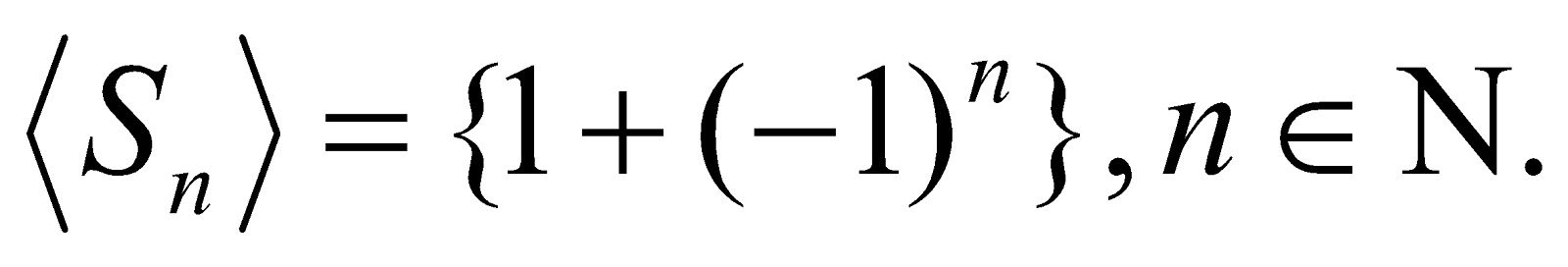1
(b) Prove that every convergent sequence is bounded. 4
(c) State and prove Cauchy’s general principle of convergence. 1+4=5
Or
Define monotonic sequence. Prove that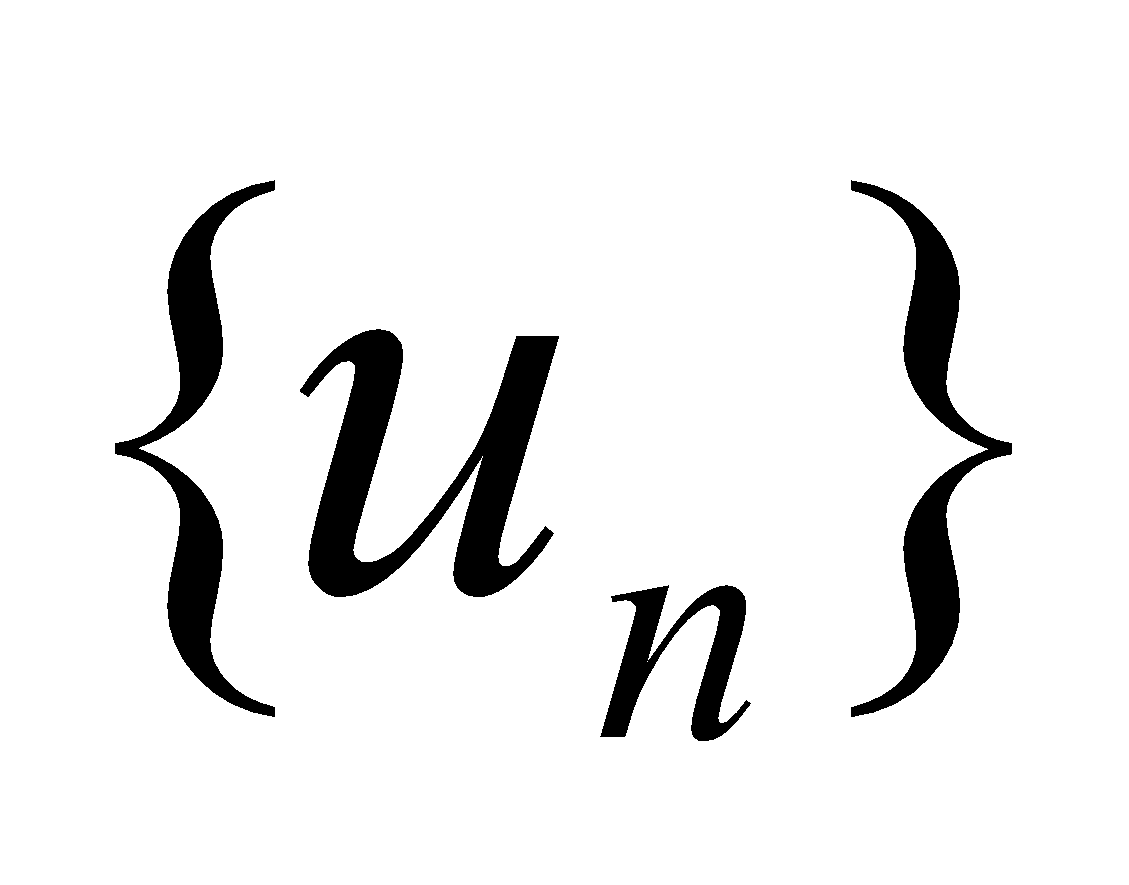is convergent, when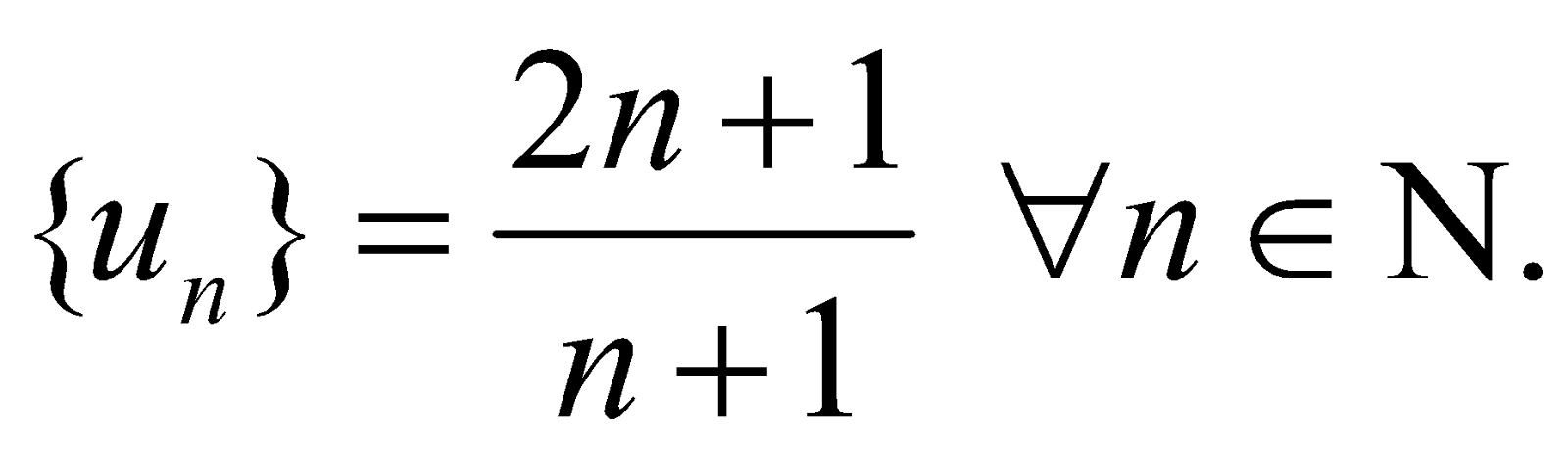2. (a) State the Cauchy’s root test for convergence.
(b) Prove that a necessary condition for a infinite series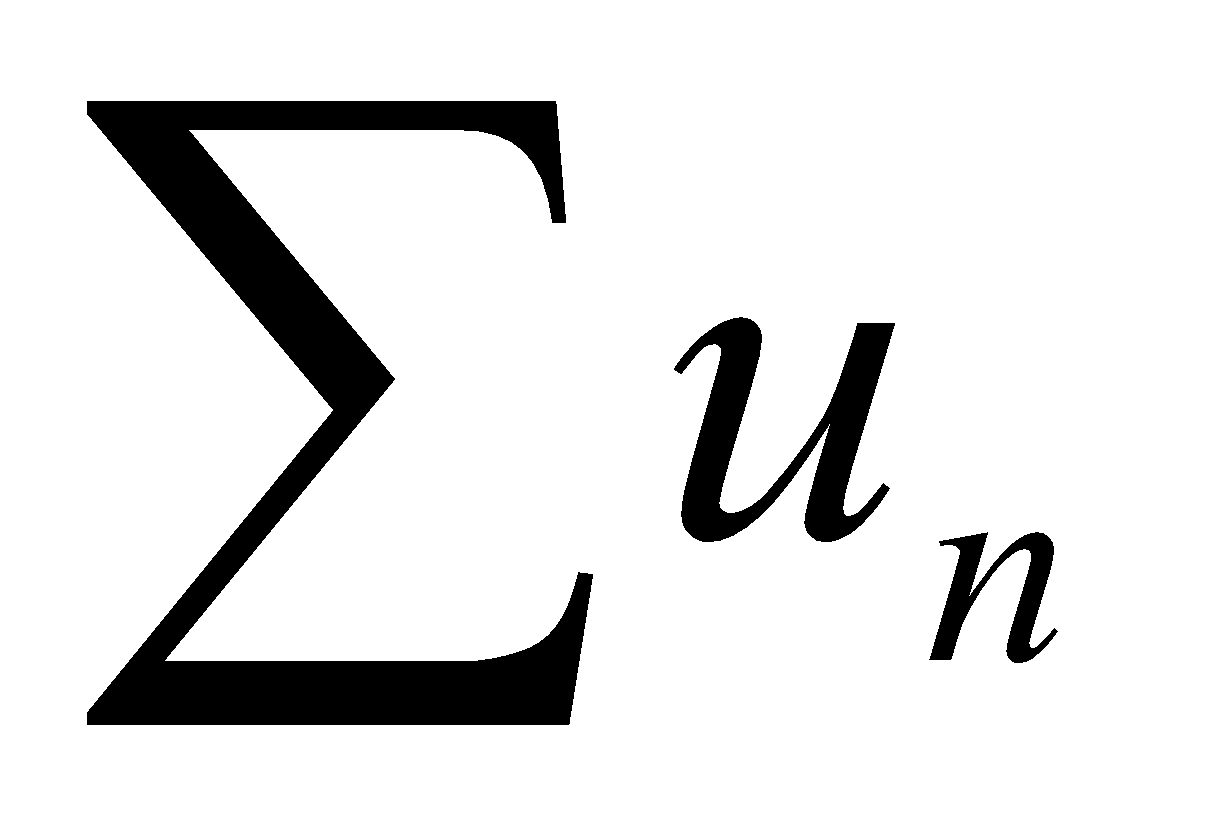to be convergent that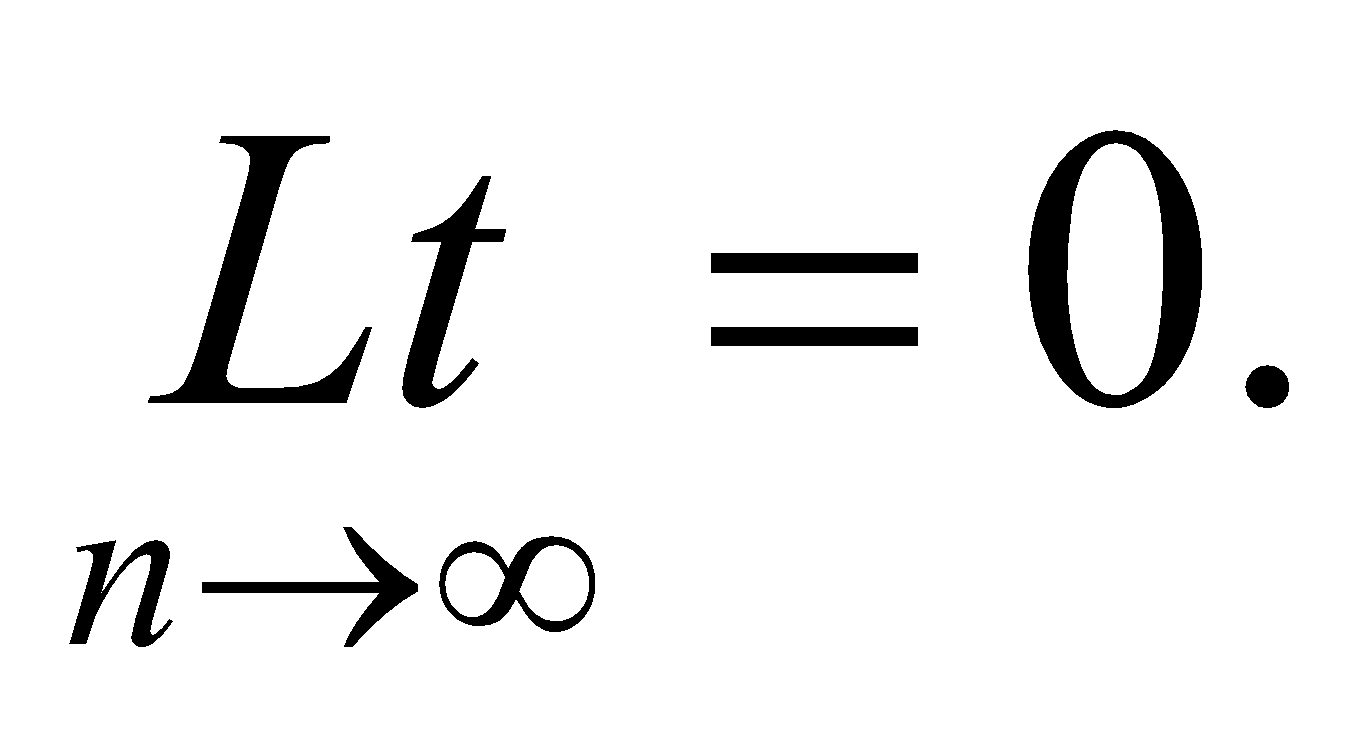(c) Test for convergence: 3
1.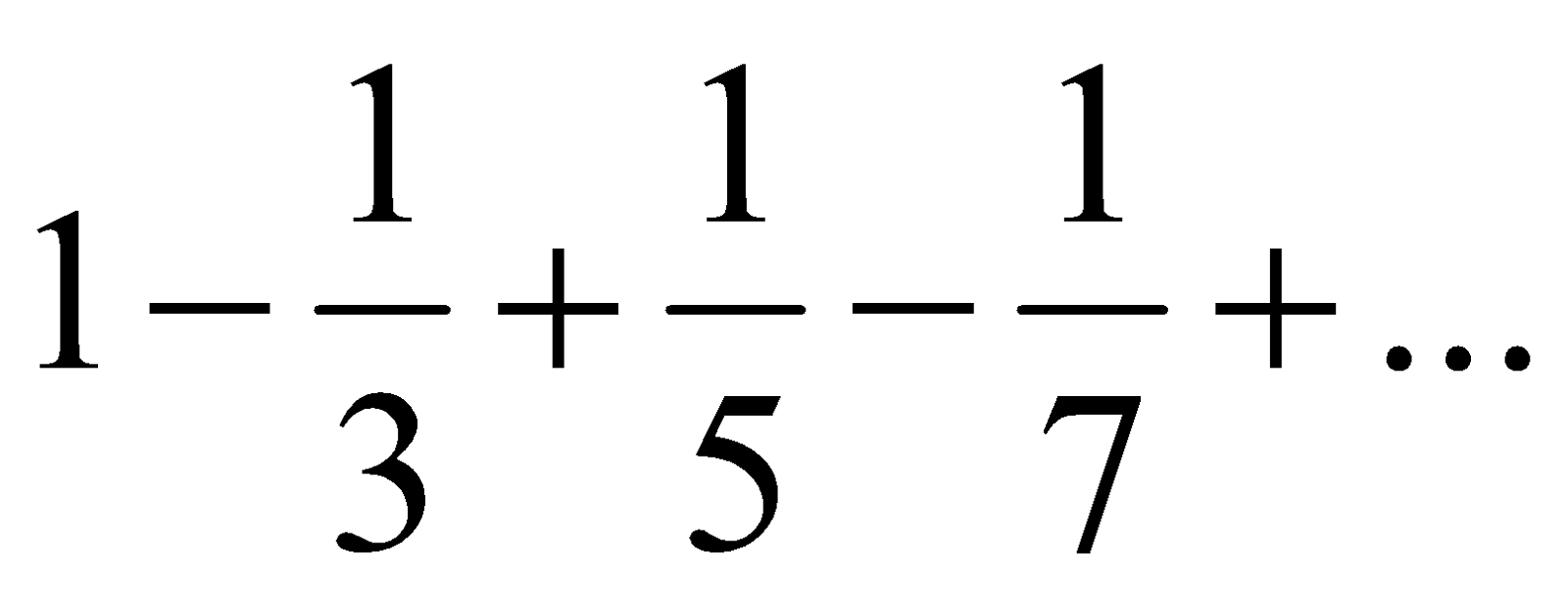2.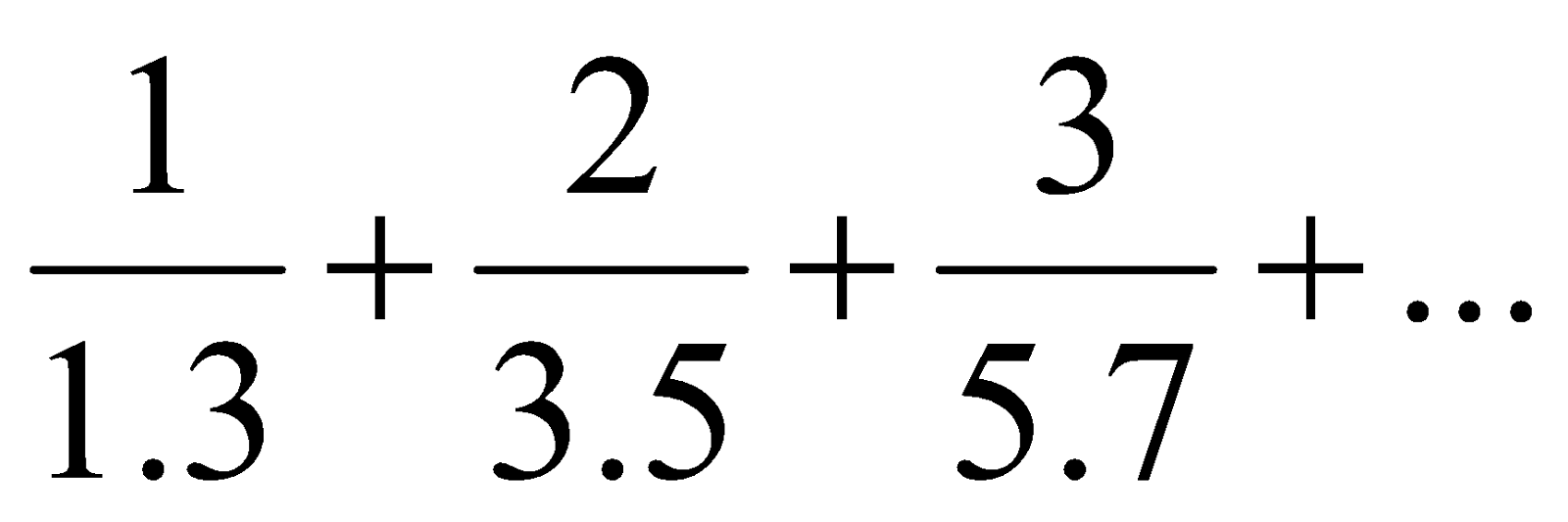(d) Discuss the convergency or divergency of the following (any one): 5
1.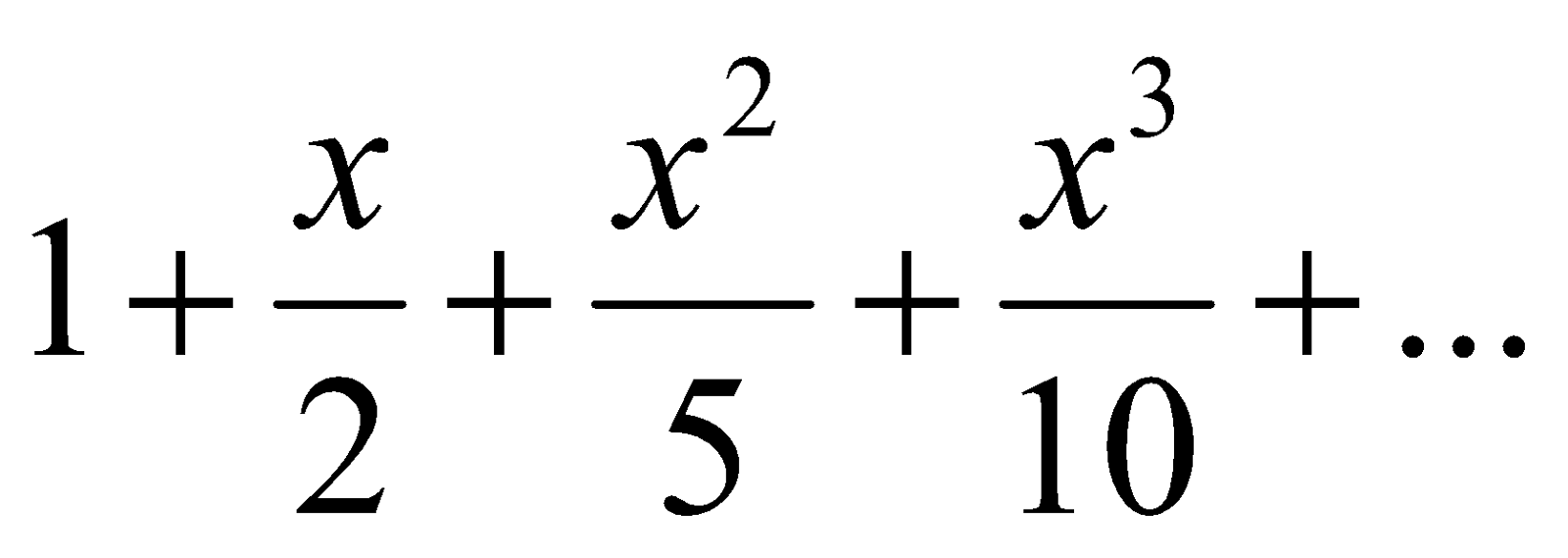2.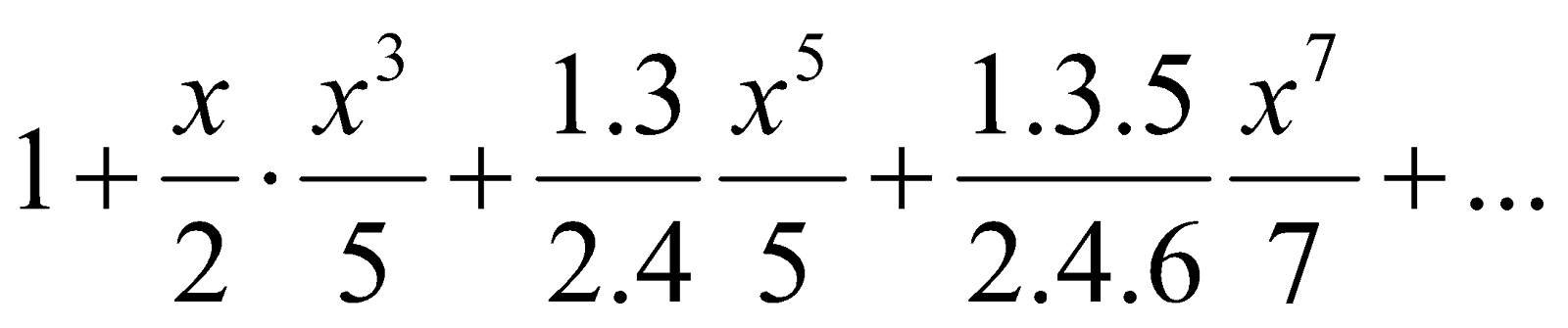3. (a) Write the fundamental theorem of algebra. 1
(b) Show that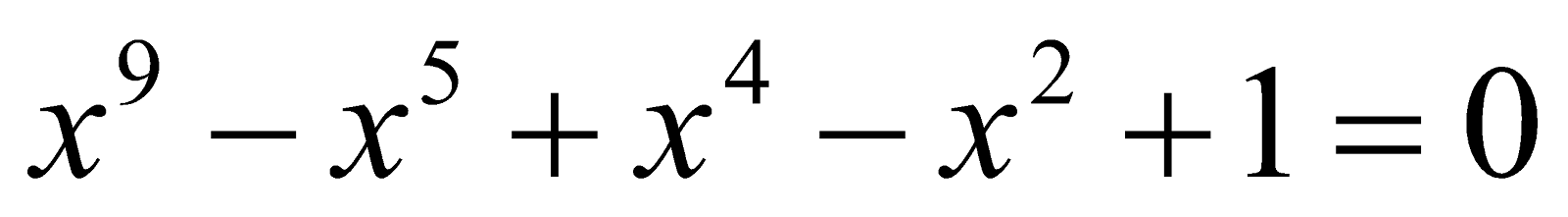has at least two imaginary roots. 2
(c) Solve the equation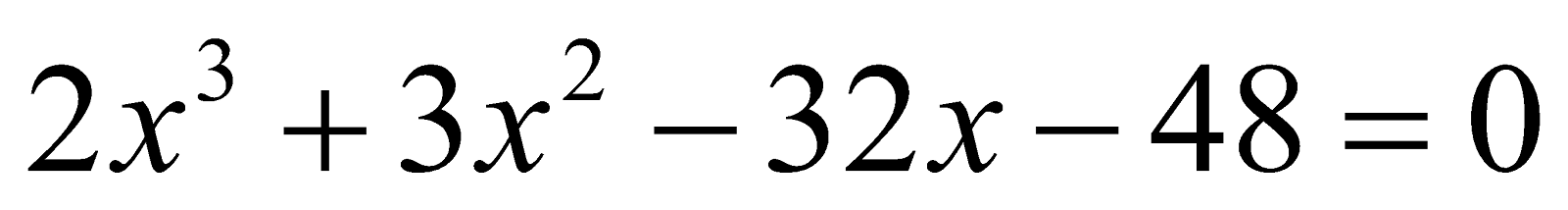Where the sum of two roots is zero 3
(d) Solve by Cardan’s method (any one):
1.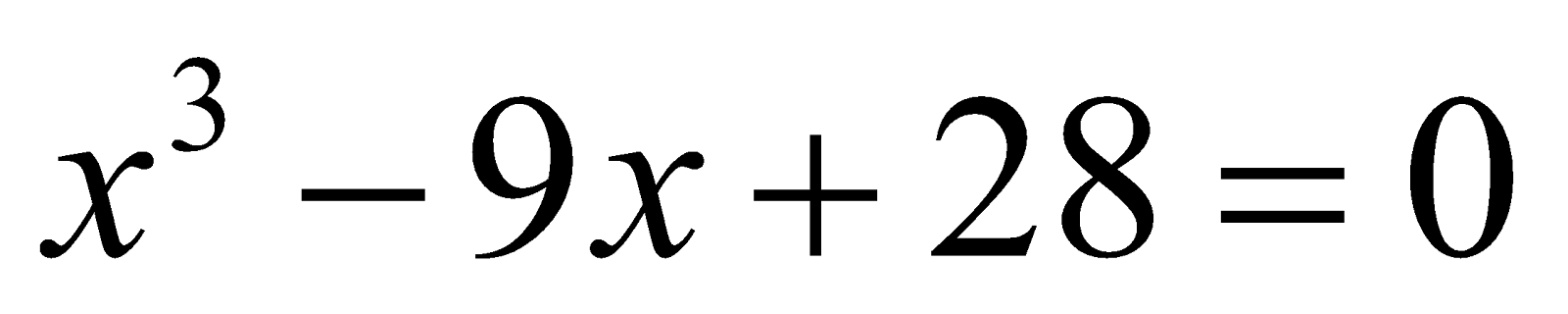2.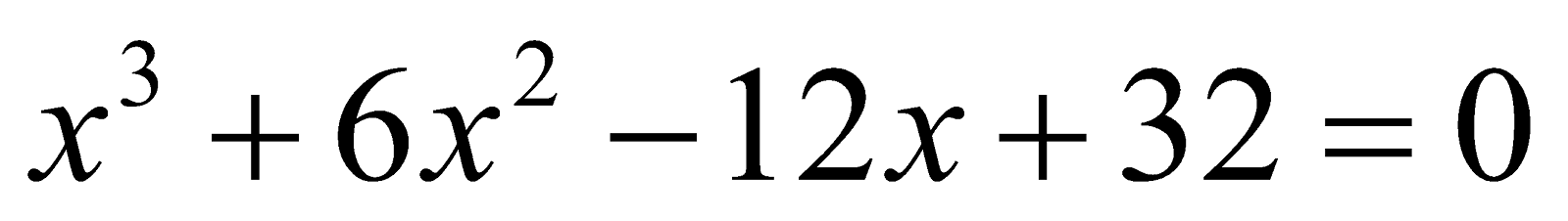(e) If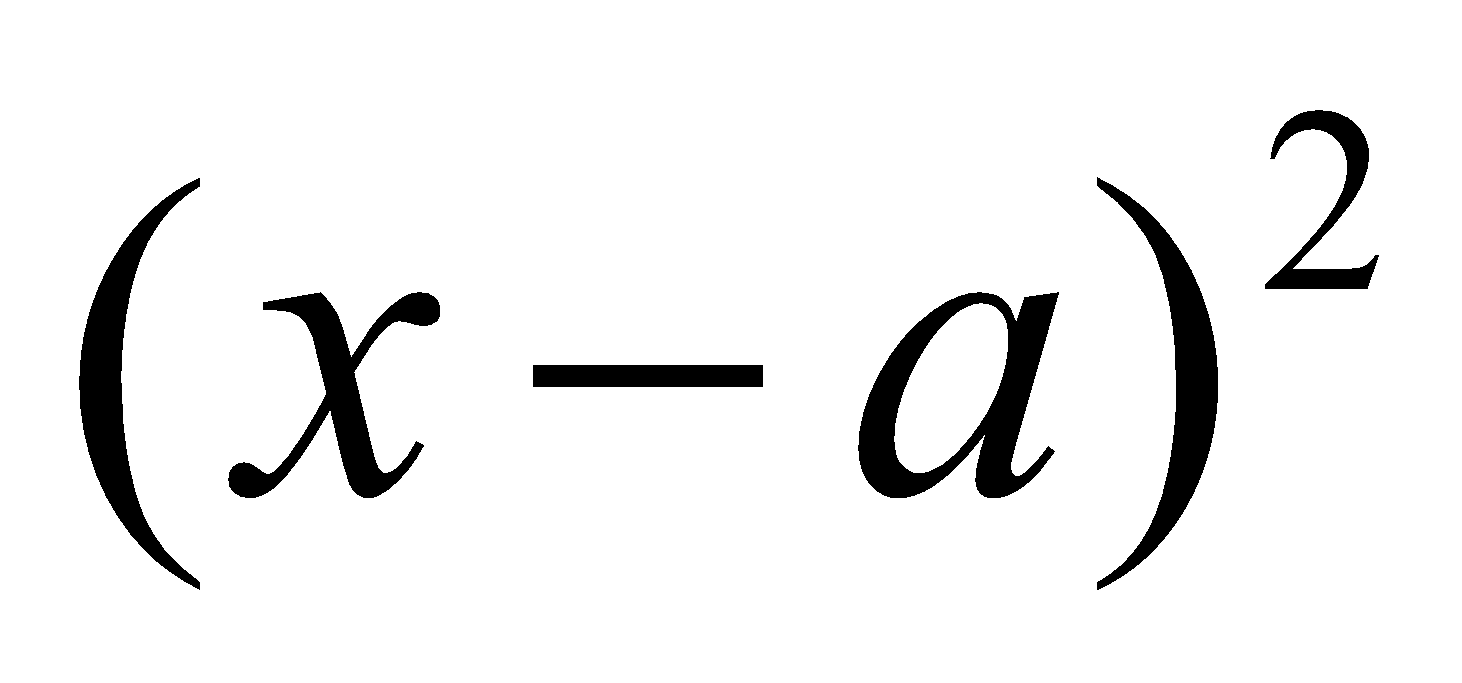is a factor of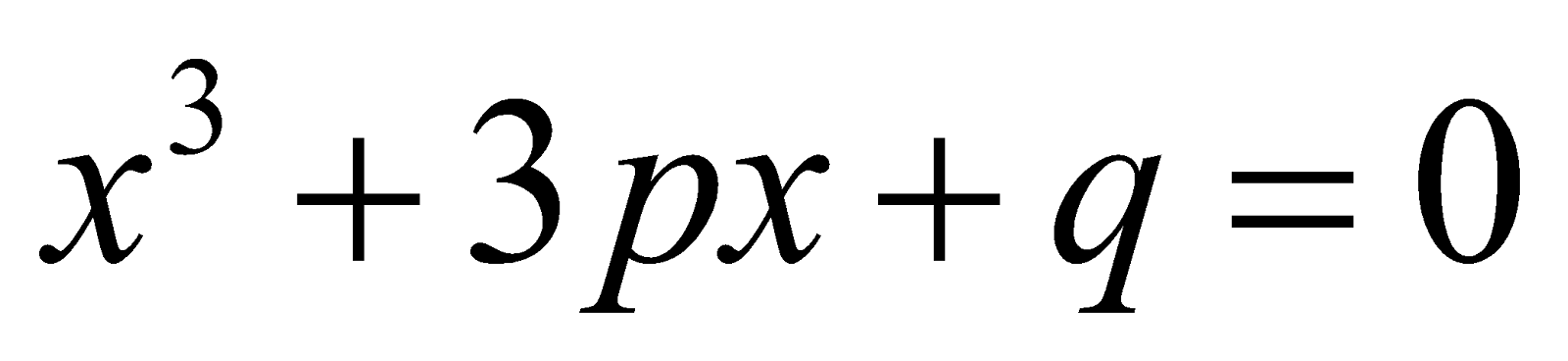then show that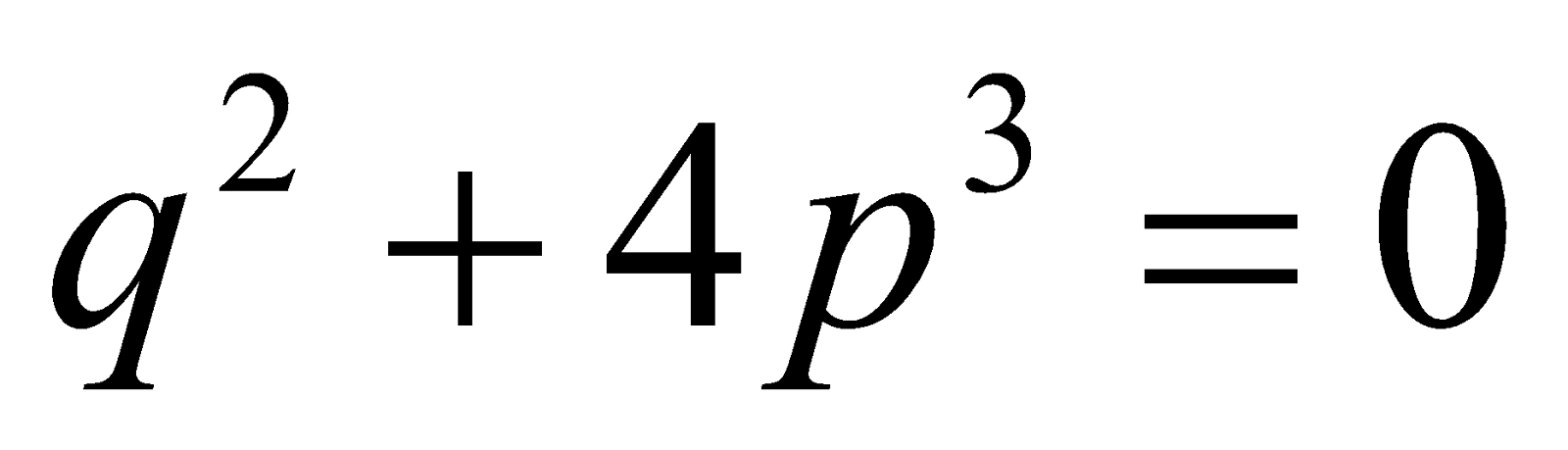GROUP – B
(Trigonometry)

4. (a) Express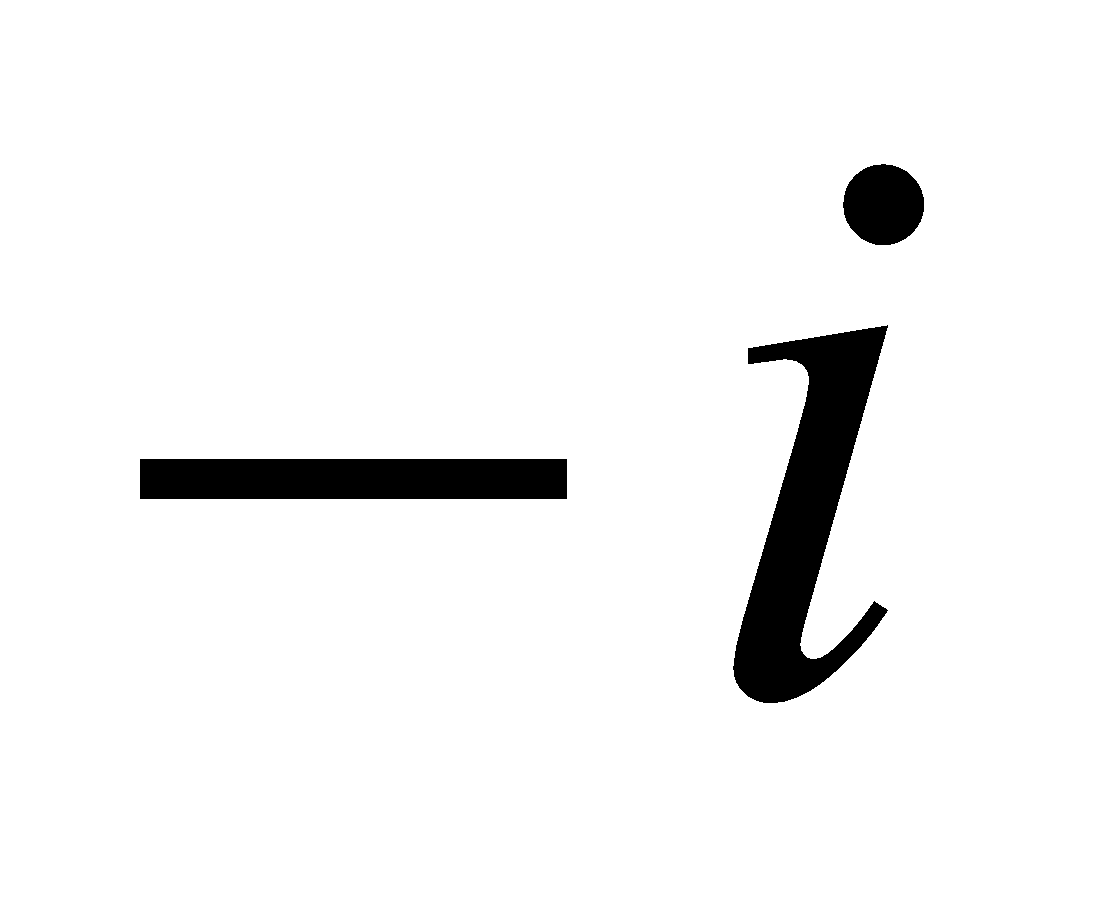in De Moivre’s form.
(b) Find the values of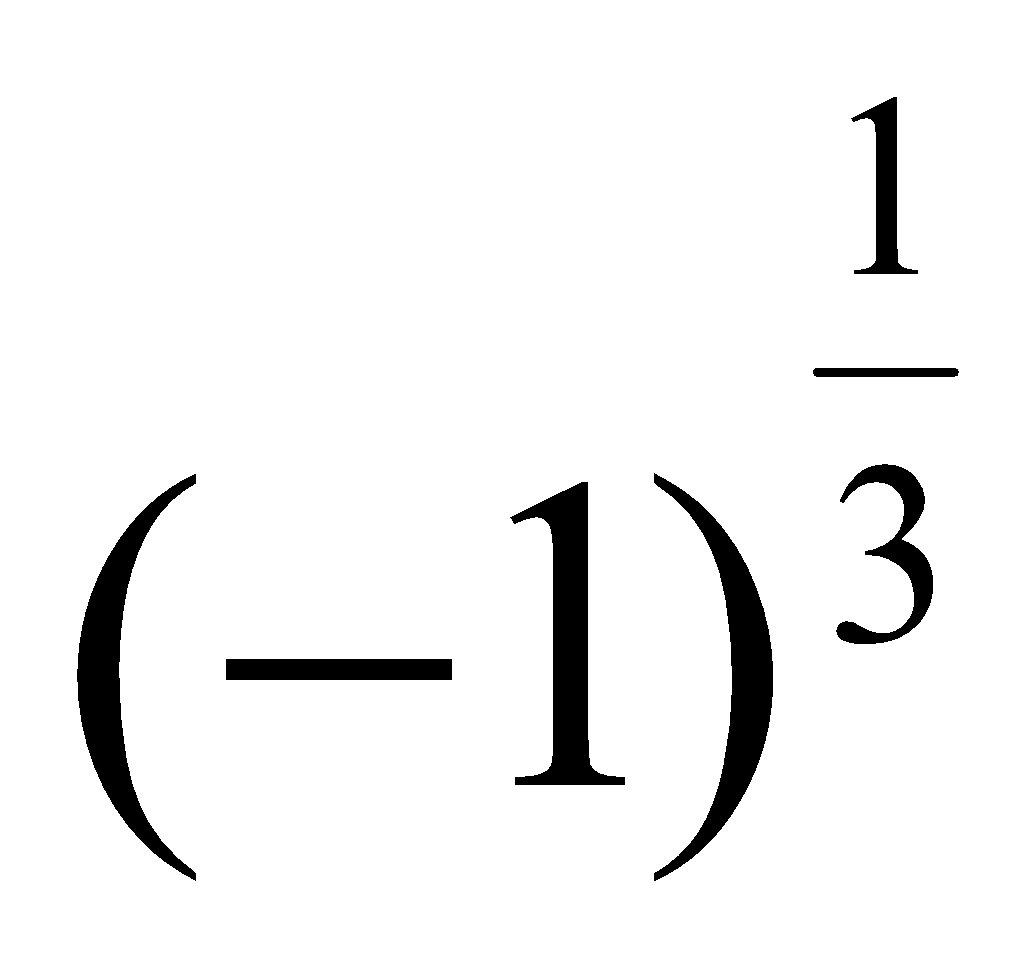.
(c) If n is a positive integer, prove thatOr
If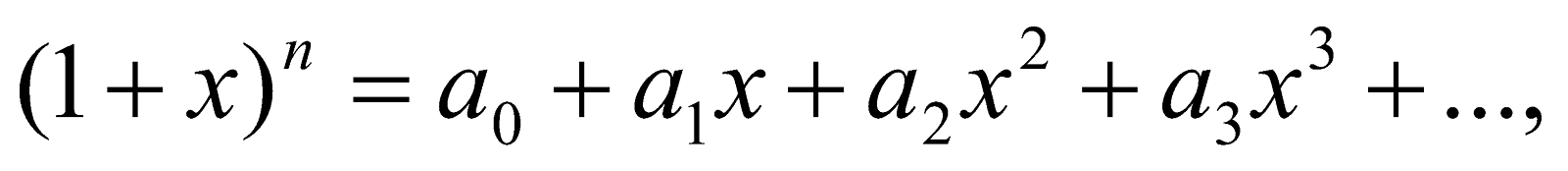then prove that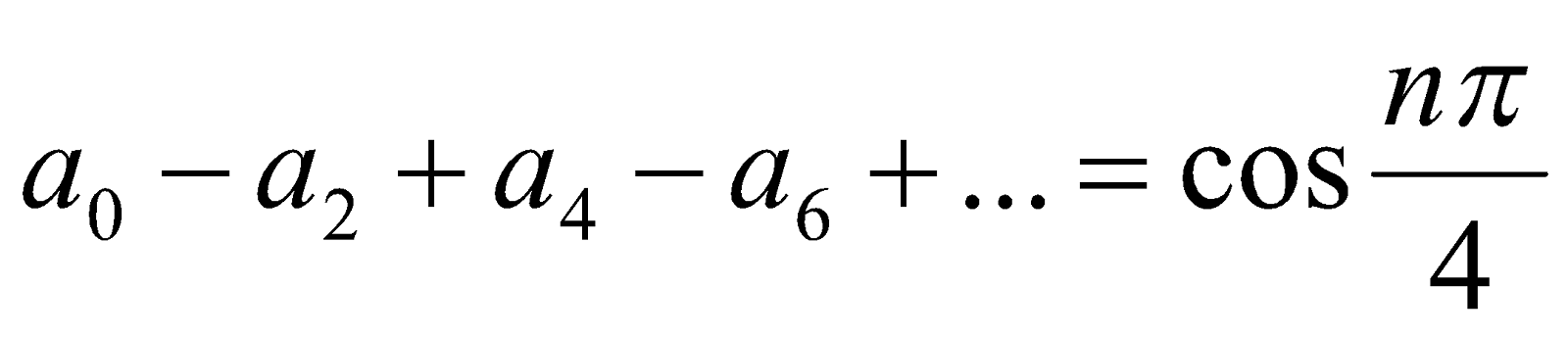.
5. If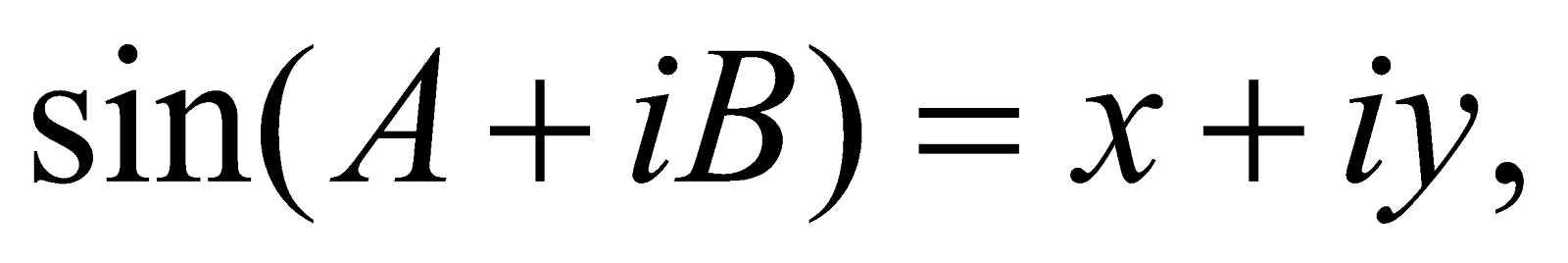then prove that 56. Prove that 47. (a) Find the sum of the series: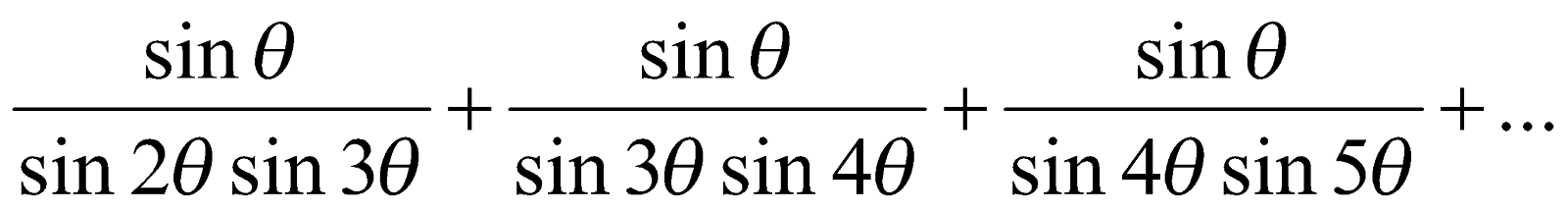(b) Find the sum of the series (any one):
1.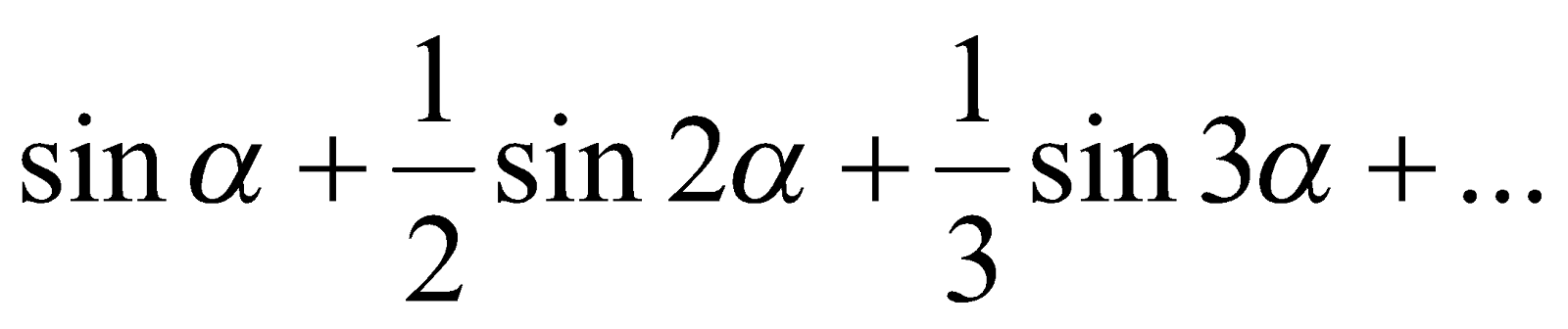2.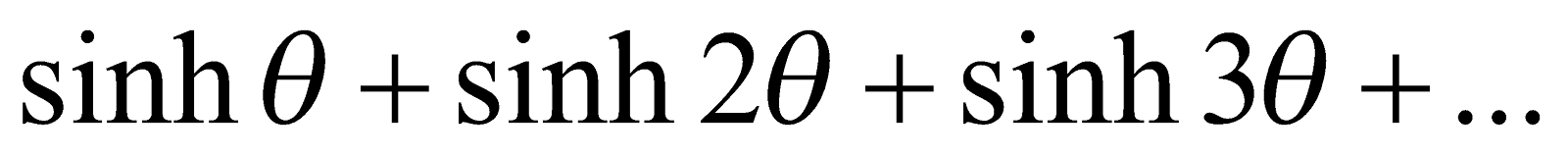GROUP – C
(Vector Calculus)
8. (a) Define space curve.
(b) A particle moves along a curve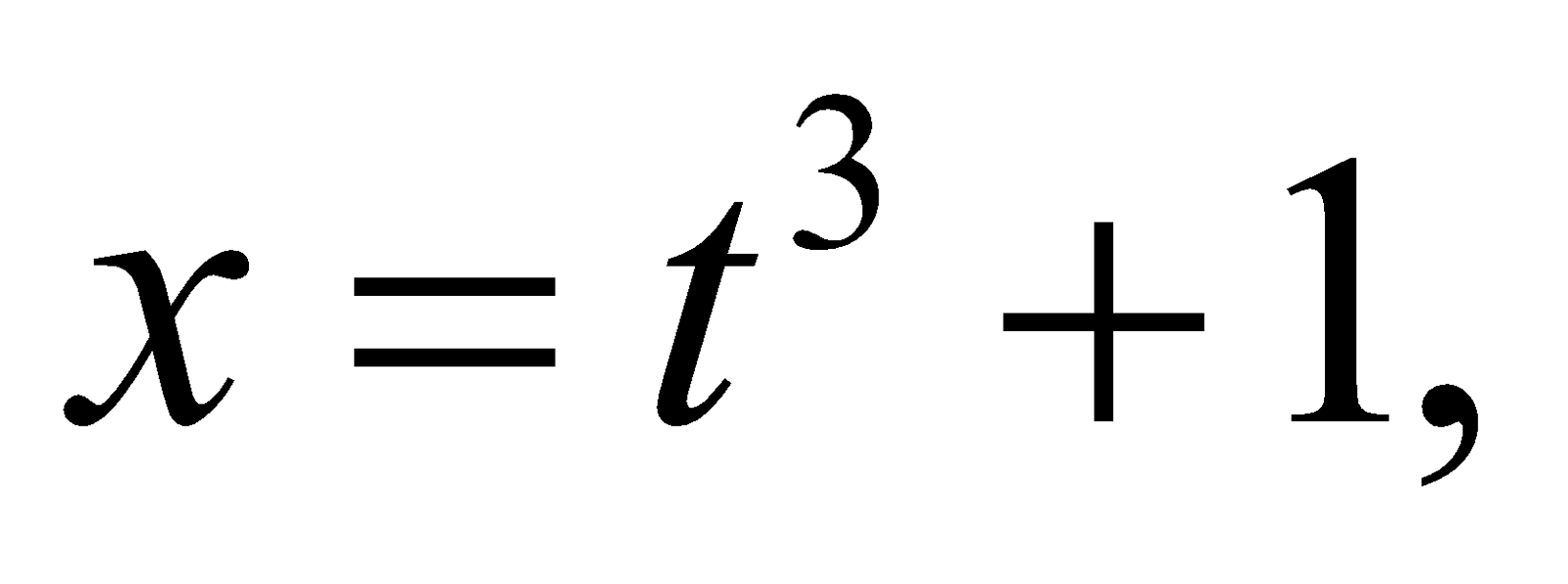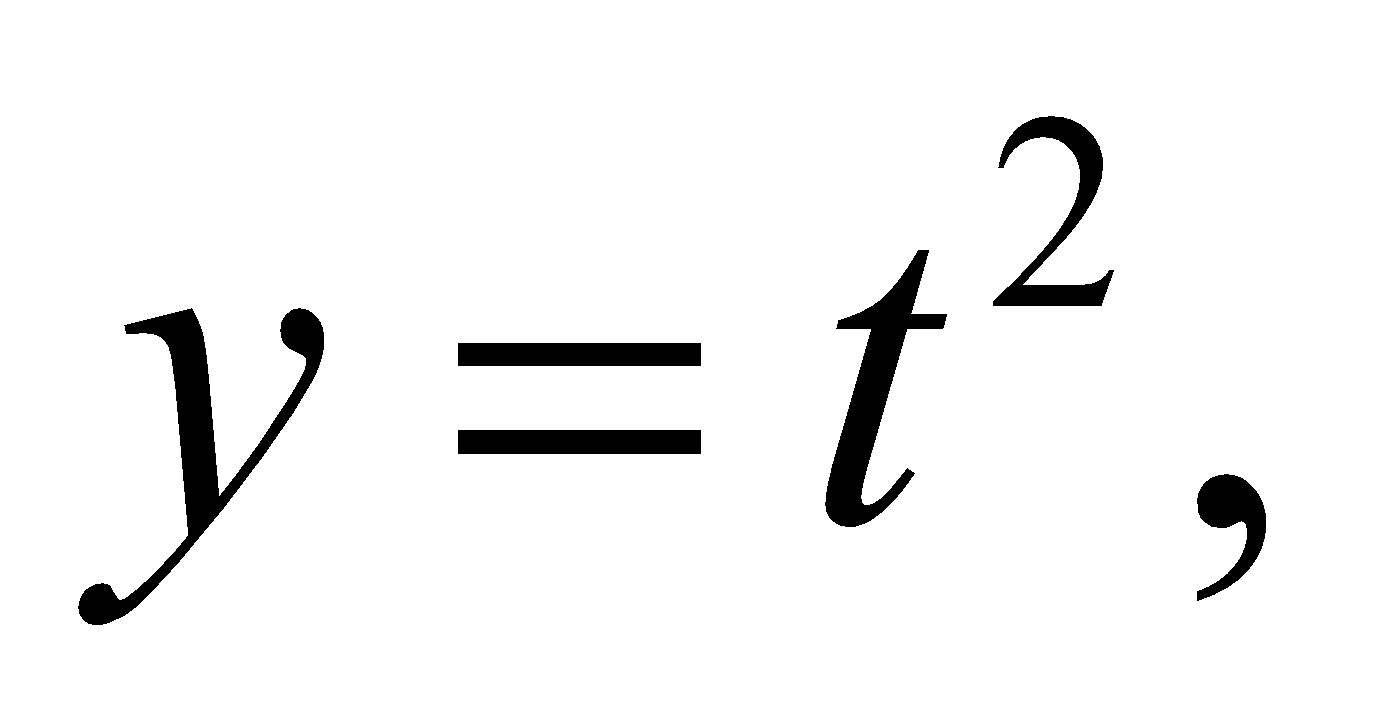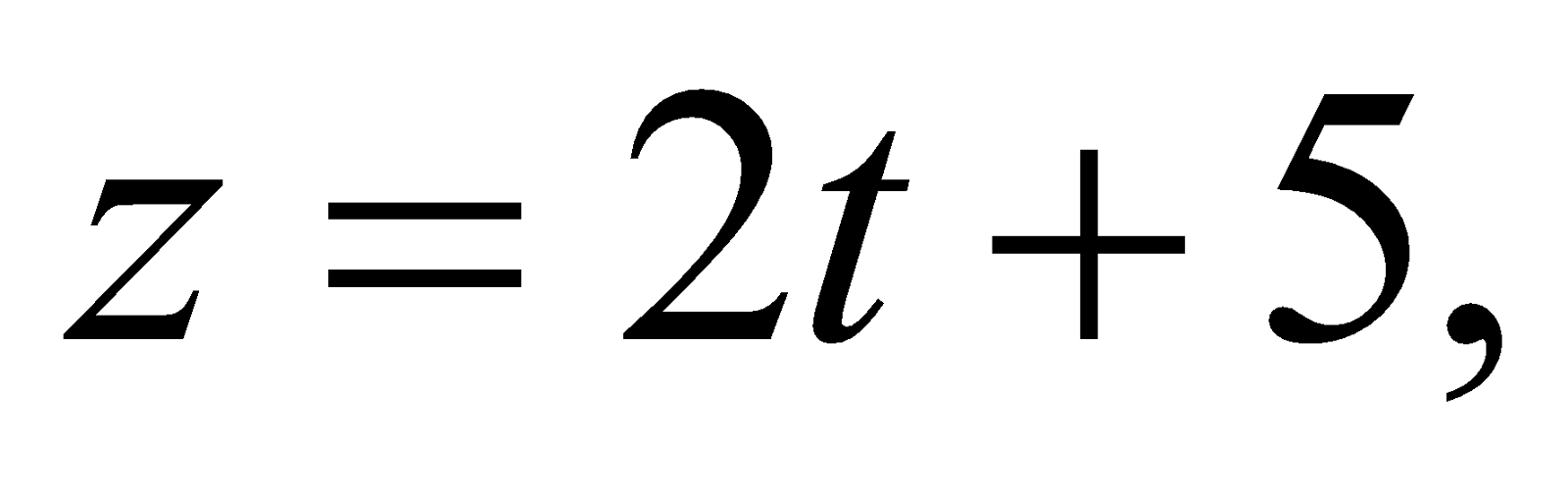wheretime is. Find the velocity and acceleration at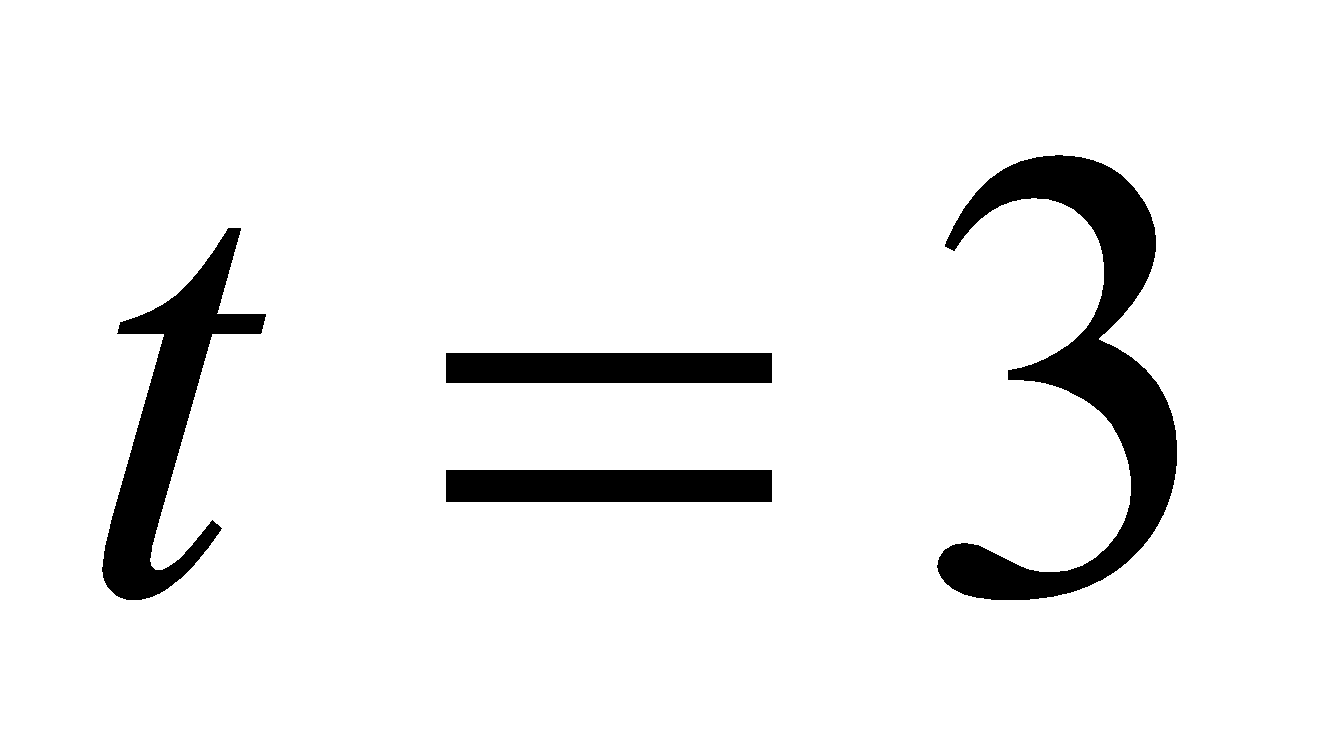(c) If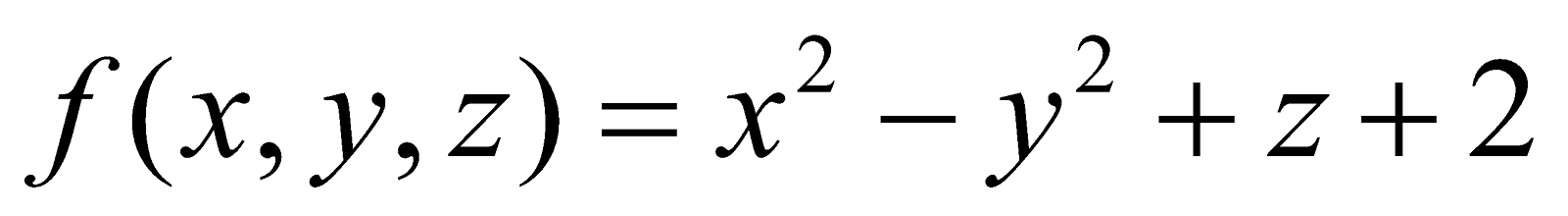, then find the unit normal vector to the surface of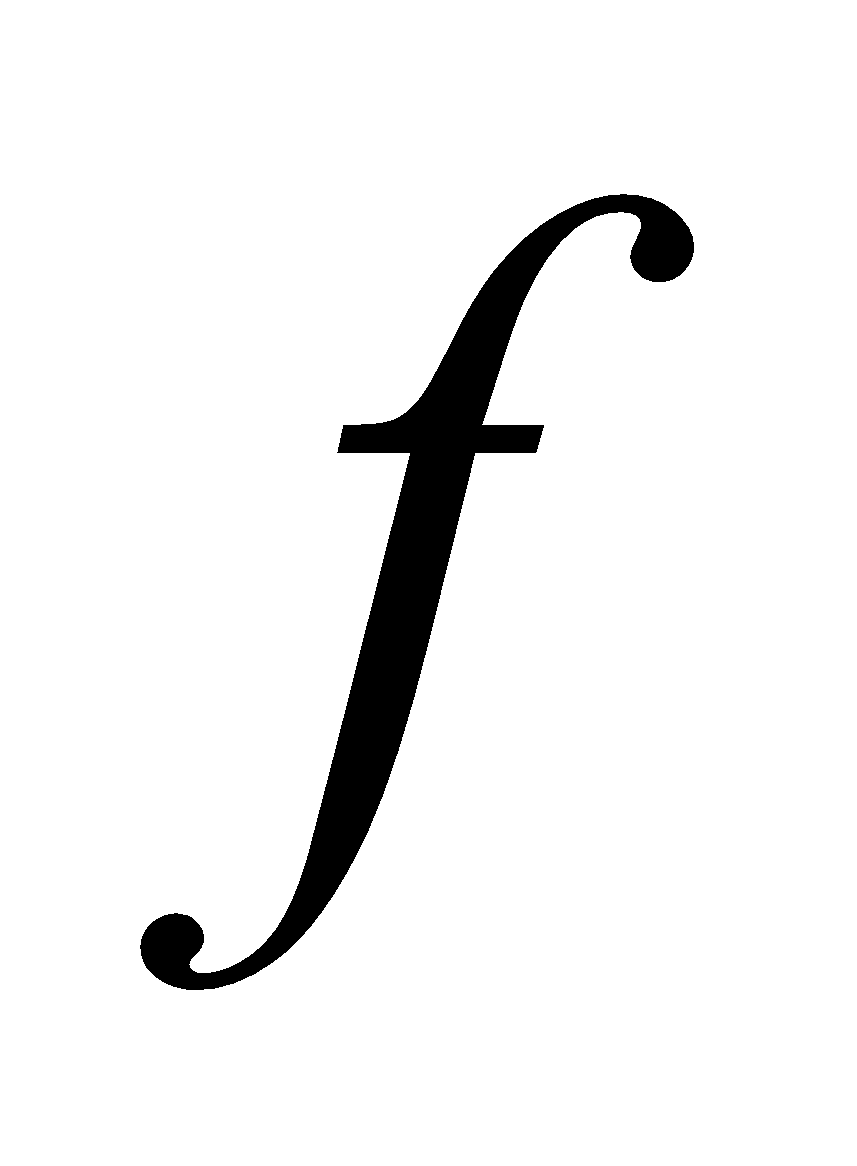at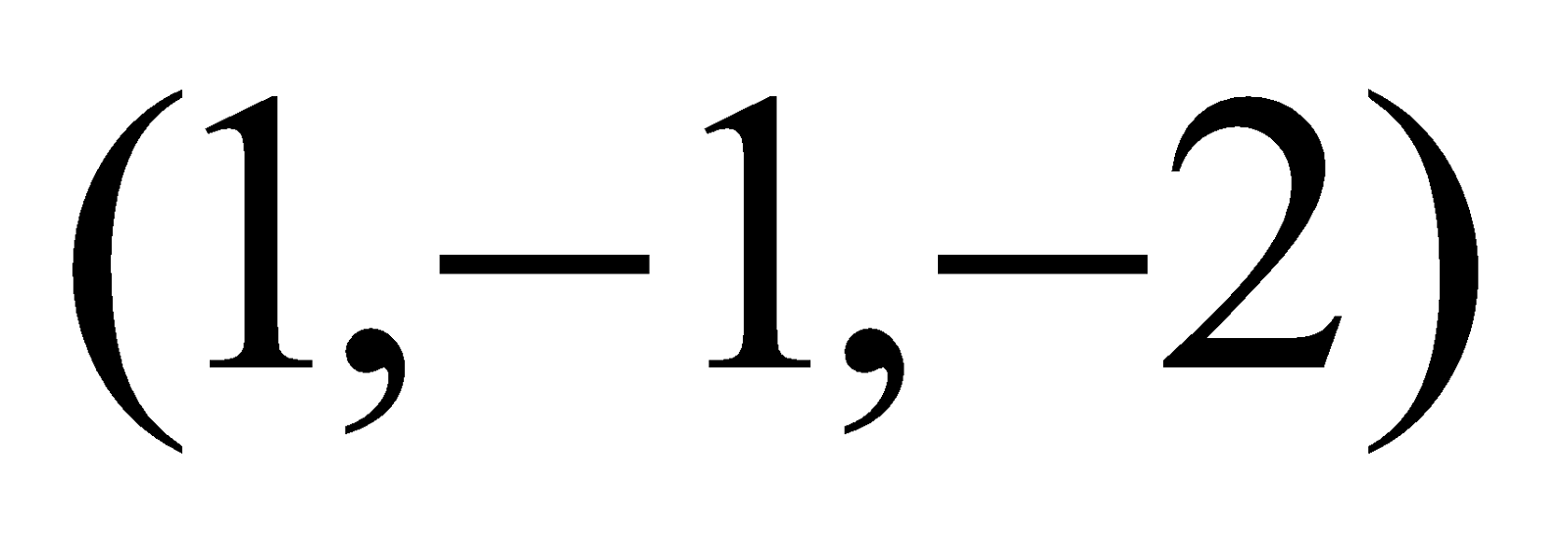.     3
(d) If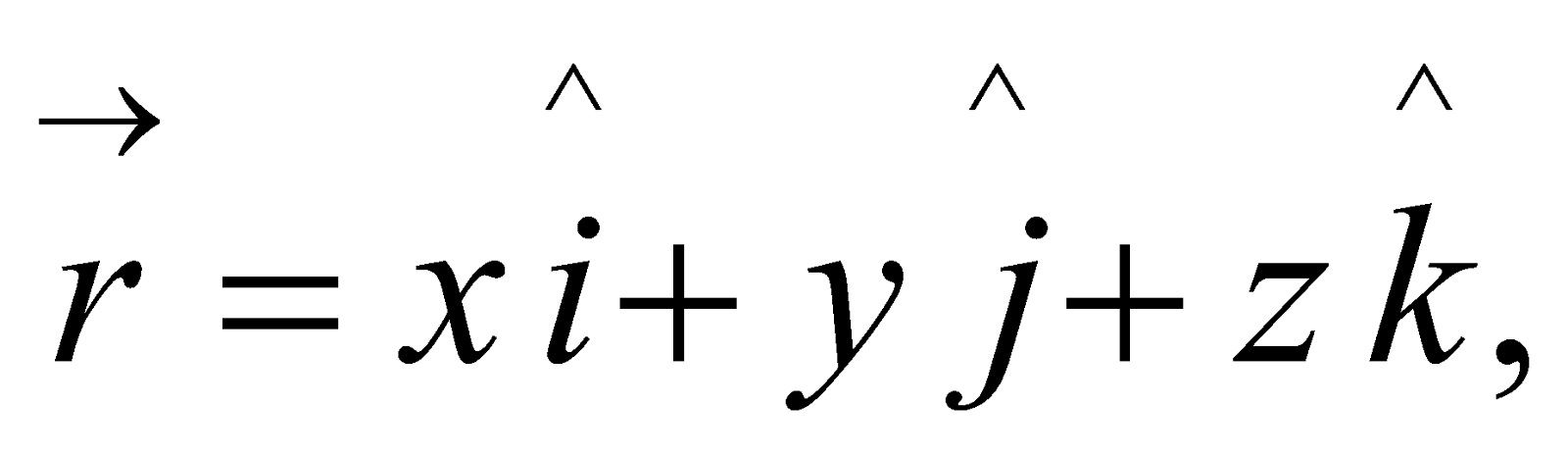then prove that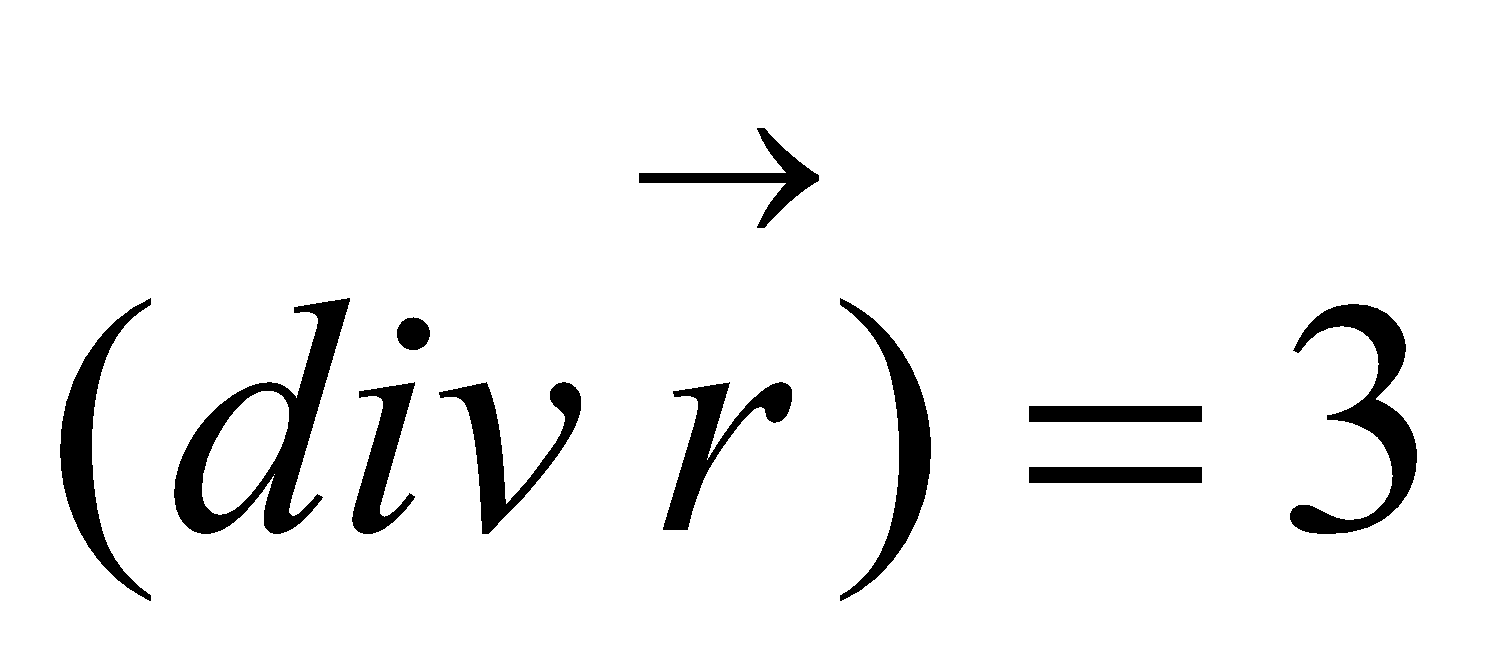2
(e) Prove any one of the following: 4
1.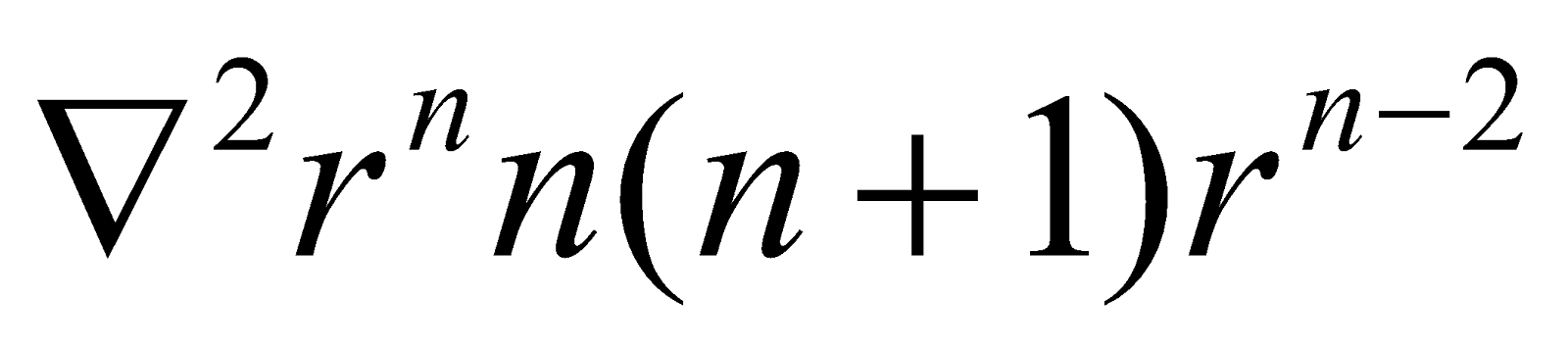2. grad(f) Prove that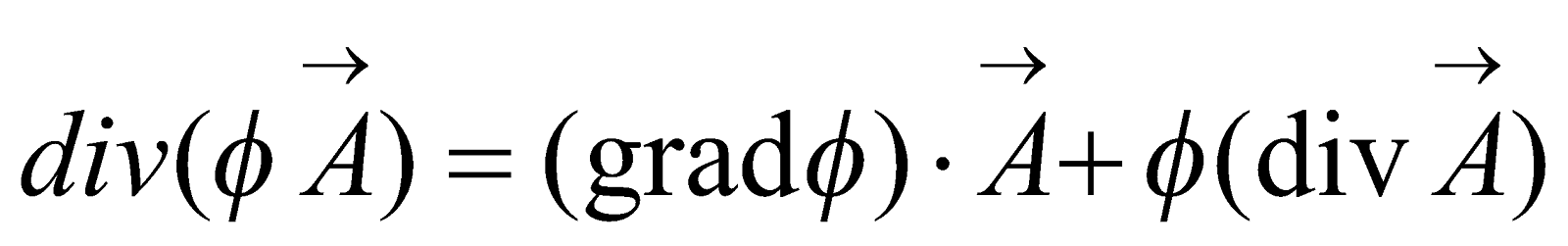3

***